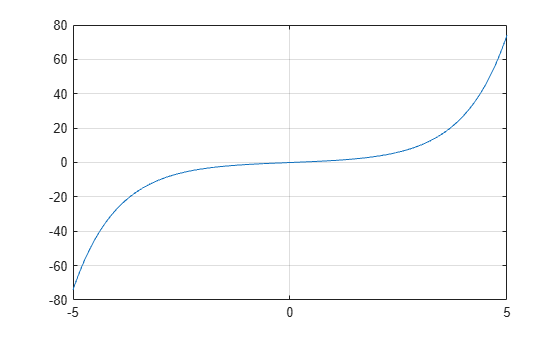sinh

Hyperbolic sine

Description

example

Y = sinh(X) returns the hyperbolic sine of the elements of X. The sinh function operates element-wise on arrays. The function accepts both real and complex inputs. All angles are in radians.

Examples

collapse all

Create a vector and calculate the hyperbolic sine of each value.

X = [0 pi 2*pi 3*pi];
Y = sinh(X)
Y = 1×4
103 ×

0    0.0115    0.2677    6.1958

Plot the hyperbolic sine over the domain $-5\le x\le 5$.

x = -5:0.01:5;
y = sinh(x);
plot(x,y)
grid onThe hyperbolic sine satisfies the identity $\mathrm{sinh}\left(\mathit{x}\right)=\frac{{\mathit{e}}^{\mathit{x}}-{\mathit{e}}^{-\mathit{x}}}{2}$. In other words, $\mathrm{sinh}\left(\mathit{x}\right)$ is half the difference of the functions ${\mathit{e}}^{\mathit{x}}$ and ${\mathit{e}}^{-\mathit{x}}$. Verify this by plotting the functions.

Create a vector of values between -3 and 3 with a step of 0.25. Calculate and plot the values of sinh(x), exp(x), and exp(-x). As expected, the sinh curve is positive where exp(x) is large, and negative where exp(-x) is large.

x = -3:0.25:3;
y1 = sinh(x);
y2 = exp(x);
y3 = exp(-x);
plot(x,y1,x,y2,x,y3)
grid on
legend('sinh(x)','exp(x)','exp(-x)','Location','bestoutside')Input Arguments

collapse all

Input angles in radians, specified as a scalar, vector, matrix, or multidimensional array.

Data Types: single | double
Complex Number Support: Yes

collapse all

Hyperbolic Sine

The hyperbolic sine of an angle x can be expressed in terms of exponential functions as

$\mathrm{sinh}\left(x\right)=\frac{{e}^{x}-{e}^{-x}}{2}.$

In terms of the traditional sine function with a complex argument, the identity is

$\mathrm{sinh}\left(x\right)=-i\mathrm{sin}\left(ix\right)\text{\hspace{0.17em}}.$